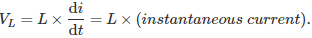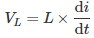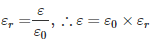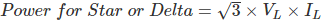# MCQs on Electric Circuits

##### Page 2 of 63. Go to page 1 2 3 4 5 6 7 8 9 10 11 12 13 14 15 16 17 18 19 20 21 22 23 24 25 26 27 28 29 30 31 32 33 34 35 36 37 38 39 40 41 42 43 44 45 46 47 48 49 50 51 52 53 54 55 56 57 58 59 60 61 62 63
01․ Instantaneous power in inductor is proportional to the
product of the instantaneous current and rate of change of current.
square of instantaneous current.
square of the rate of change of current.
temperature of the inductor.

The instantaneous voltage across the inductor is expressed as the product of inductance and rate of change of current through it. Power is expressed as the product of current and voltage. Hence instantaneous power in an inductor is proportional to the product of instantaneous current and rate of change of current through it.02․ The voltage induced in an inductor is represented as,
product of its inductance and current through it.
ratio of its inductance to current through it.
ratio of current through it to its inductance.
product of its inductance and rate of change of current through it.

The instantaneous voltage across the inductor is expressed as the product of inductance and a rate of change of current through it as it doesn't allow any certain change of current through it. Voltage induced in an inductor is written as03․ Absolute permittivity of dielectric medium is represented as
ε0
εr
εr0
εrε0

The relative permittivity (εr) of the medium is defined as the ratio of actual permittivity of the medium to the absolute permittivity of air or vacuum. Absolute permittivity of air or vacuum is expressed as ε0. Hence the absolute permittivity of dielectric medium is represented as the product of relative permittivity of the medium and absolute permittivity of air or vacuum.04․ Magnetic flux has the unit of
Newton
Ampere turn
Weber
Tesla

The unit of magnetic flux is called Weber in honor of famous scientist Wilhelm Eduard Weber (1804 – 1891). Magnetic flux has also another unit named Maxwell. 1 Maxwell = 10-8 Weber.

05․ If all the elements in a particular network are linear, then the superposition theorem would hold, when the excitation is
DC only
AC only
Either AC or DC
An Impulse

Superposition theorem can be applied for both AC as well DC excitation to calculate the voltage or current calculations. It holds for both DC and AC excitation, if the circuit is linear. But superposition theorem is not applicable for power calculations.

06․ In balanced bridge, if the positions of detector and source are interchanged, the bridge will still remain balanced. This can be explained from which theoem
Reciprocity theorem
Thevinin's theorem
Norton's theorem
Compensation theorem

When response to excitation is constant even though we interchange the excitation and responses then the reciprocity theorem is verified for the given network.

07․ If P is the power of a star connected system then what will be power of an equivalent delta connected system?
P
3P
P/3
None of the above

The power in both of the connections is same either it is connected in Star or Delta.08․ Which of the followings is/are active element?
Voltage source
Current source
Both
None of these.

Active elements are capable of delivering energy independently for long time or ideally infinite time. Both voltage and current source are active element and they can change energy level of a circuit.

09․ Which of the following are the passive elements?
Resistor
Bulb
Both
None of these.

When the element is not capable of delivering energy independently, are called as passive element. Both resistor and bulb are the passive elements and they can't increase the energy level of a circuit.

10․ Power dissipation in ideal inductor is
Maximum
Minimum
Zero
A finite value

Internal resistance of ideal inductor is zero. As there is no resistance, there should not be any dissipation of power. Pure inductor has no resistive component, so it active power dissipation is zero.

<<<1234>>>